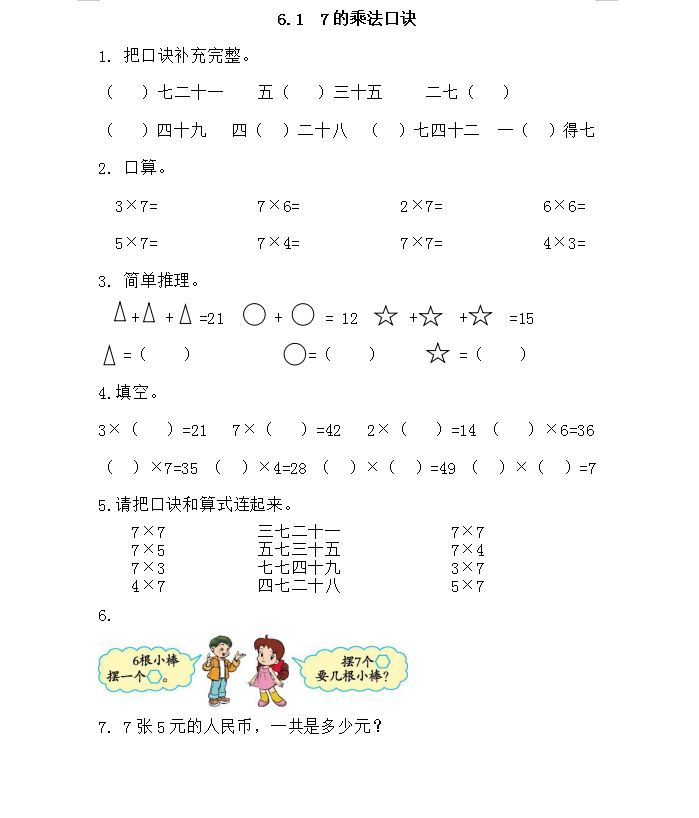6.1  7的乘法口诀

1. 把口诀补充完整。

（   ）七二十一    五（   ）三十五     二七（   ）

（   ）四十九   四（  ）二十八  （  ）七四十二  一（  ）得七

1. 口算。

3×7=            7×6=            2×7=            6×6=

5×7=            7×4=            7×7=            4×3=